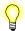# ilogb(), ilogbf()

Compute the integral part of a logarithm

## Synopsis:

```#include <math.h>

int ilogb ( double x );

int ilogbf (float x );```

## Arguments:

x
The number you want to compute the integral part of the logarithm.

## Library:

libm

Use the -l m option to qcc to link against this library.

## Description:

The ilogb() and ilogbf() functions compute the integral part of:

logr |x|

as a signed integral value, for nonzero finite x, where r is the radix of the machine's floating point arithmetic.

## Returns:

The exponent part of x, in integer format:

If x is: ilogb() returns:
0 -INT_MAX
NAN INT_MAX
Negative infinity INT_MAX
Positive infinity INT_MAXIf an error occurs, these functions return 0, but this is also a valid mathematical result. If you want to check for errors, set errno to 0, call the function, and then check errno again. These functions don't change errno if no errors occurred.

## Examples:

```#include <stdio.h>
#include <stdlib.h>
#include <math.h>

int main( void )
{
printf( "%f\n", ilogb(.5) );

return EXIT_SUCCESS;
}```

## Classification:

Safety:
Cancellation point No
Interrupt handler No
Signal handler No